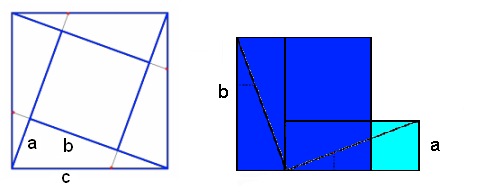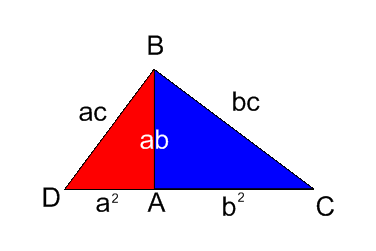#### You may also like### Doodles

Draw a 'doodle' - a closed intersecting curve drawn without taking pencil from paper. What can you prove about the intersections?### Russian Cubes

I want some cubes painted with three blue faces and three red faces. How many different cubes can be painted like that?### Picture Story

Can you see how this picture illustrates the formula for the sum of the first six cube numbers?

# Pythagoras Proofs

##### Age 14 to 16 Challenge Level:

Herschel of the European School of Varese, Italy and Patrick of Woodbridge School both sent us in their ideas for the first proof.We rearrange the construction on the left into the L shape on the right as shown above. Each of the four triangles are congruent and have side lengths $a$ and $b$ and hypotenuse $c$. Clearly the area of the original square is $c^2$. After rearranging the shapes we find that the dark blue square has sides of length $b$ and the light blue square has sides of length $a$. The total area of the L shape must therefore be $a^2 + b^2$. Hence $a^2 + b^2 = c^2$.

This is a good start and explains the ideas nicely. However there are a few details which you may want to clarify. How do we know the triangles are right angled triangles? How do we know that the L shape on the right is definitely made up of two squares i.e. how do we know it isn't made up of two rectangles?

Herschel and Patrick then continued with the second proof:

\eqalign{ \mbox{Area of Square} &= (a+b)^2\cr &= {{a^2 + 2ab + b^2}}} Area of the Trapezium = Area of square divided by 2 (rotational symmetry) $$\mbox{Area of Trapezium} = {{a^2 + 2ab + b^2}\over{2}}$$ We can also think of the area of the Trapezium as the sum of areas of the three triangles. So: \eqalign{ \mbox{Area of Trapezium} &= {{ab\over{2}}+ {ab\over{2}}+ {c^2\over{2}}}\cr &={{ab + ab + c^2\over{2}}}\cr &= {{2ab + c^2\over{2}} }}
Hence\eqalign{ {{2ab + c^2\over{2}} } &= {{a^2 + 2ab + b^2}\over{2}}\cr 2ab+c^2 &= a^2+2ab+c^2\cr c^2 &= a^2 + b^2}

Herschel: "This proof was the simplest"
Patrick: "I find this proof the most interesting, and probably easier to explain. I was quite surprised to find Pythagoras's Theorem emerging from the formulae."

Andrew from Island School sent us his work on the third proof.For the red triangle, $DA = a^2$, $DB=ac$ and $AB=ab$.
For the blue triangle, $AB=ab$, $AC=b^2$ and $BC=bc$.
For the combined triangle, $DB=ac$ and $BC=bc$.
To get the missing length which is $DC$, the enlargement has the scale factor of $c$. Then the sides of the triangle with the scale factor of $c$ would be $ca$, $cb$ and $c^2$.
But $DC=DA+AC$, so $a^2+b^2=c^2$.

Good work, Andrew!